tzyt的博客 2022-12-28T22:15:45.817Z https://ttzytt.com/ tzyt Hexo [Stanford CS144] Lab0-Lab3 实验记录 https://ttzytt.com/2022/12/CS144_lab0-3_rec/ 2022-12-25T05:00:00.000Z 2022-12-28T22:15:45.817Z

# Lab0 networking warmup

Lab 要求实现一个在内存层面上可靠的字节流（ByteStream），感觉和 unix 中的管道挺像的。其实这样先进先出的结构完全可以直接使用 STL 的 queue<char> 实现，会非常简单。但是考虑到 lab 的要求是一个固定大小（capacity）的字节流，个人认为直接开个数组模拟更合适，速度也应该会更快。

string ByteStream::peek_output(const size_t len) const {    size_t peek_size = min(buffer_size(), len);    size_t i = 0;    string ret = "";    ret.resize(peek_size);    while (i < peek_size) {        ret[i] = _data[(_head + i) % _capa];        i++;    }    return ret;}

[100%] Testing Lab 0...Test project /mnt/e/ocourses/st_cs144/sponge/build    Start 26: t_byte_stream_construction1/9 Test #26: t_byte_stream_construction .......   Passed    0.01 sec    Start 27: t_byte_stream_one_write2/9 Test #27: t_byte_stream_one_write ..........   Passed    0.01 sec    Start 28: t_byte_stream_two_writes3/9 Test #28: t_byte_stream_two_writes .........   Passed    0.01 sec    Start 29: t_byte_stream_capacity4/9 Test #29: t_byte_stream_capacity ...........   Passed    0.22 sec    Start 30: t_byte_stream_many_writes5/9 Test #30: t_byte_stream_many_writes ........   Passed    0.01 sec    Start 31: t_webget6/9 Test #31: t_webget .........................   Passed    0.81 sec    Start 53: t_address_dt7/9 Test #53: t_address_dt .....................   Passed    0.05 sec    Start 54: t_parser_dt8/9 Test #54: t_parser_dt ......................   Passed    0.01 sec    Start 55: t_socket_dt9/9 Test #55: t_socket_dt ......................   Passed    0.01 sec100% tests passed, 0 tests failed out of 9Total Test time (real) =   1.17 sec[100%] Built target check_lab0

# Lab1 stitching substring into a byte bytestream

## 实现

### 需求

• 求出和一个 Seg 的交集，即前面提到的 $u \cap x$ 操作。
• 删去一个 Seg，即前面提到的 $u = u - (u \cap x)$ 操作。

         1        2(c1)            3(cn)            4Segs a : |---|    |-----|          |--------|       |---|Seg  b :        |-----------------------|

### 算法

template <integral T, bool REC_LEN>typename std::pair<typename Segs<T, REC_LEN>::s_iter_t, typename Segs<T, REC_LEN>::s_iter_t>Segs<T, REC_LEN>::intersect_iter(const Seg<T> &b) const {    // return the first and last iterator of the intersected segments    // 返回第一个和最后一个和 b 有重叠的段的迭代器    if (b.len() == 0)        return {_segs.end(), _segs.end()};    auto fir = fir_GT_iter_r(b.l); // 前文讲的 c1，是第一个右端点比查询段大的段    if (fir != _segs.end() && ((*fir) ^ b).len() == 0)  // if no intersection        fir = _segs.end();    auto las = lst_LT_iter(b.r); // 前文的 cn，是最后一个左端点比查询段的右端点小的段    if (las != _segs.end() && ((*las) ^ b).len() == 0)        las = _segs.end();    // 处理 c1 和 cn 没找到的一些情况    if (fir == _segs.end() && las != _segs.end())        fir = las;    if (fir != _segs.end() && las == _segs.end())        las = fir;    return {fir, las};}

……    // insert new arrival into _tmp    const Seg coverage{index, index + data.size()}; // 新到达数据段的范围    auto &&unfilled_intersect = _unfilled_segs ^ coverage; // 这里我重载了 ^ 这个符号，表示求交    for (auto &s : unfilled_intersect) {        // s 表示一个未填充的段        for (size_t i = s.l; i < s.r && (i - _fir_unpushed_idx) <= _capacity; i++) {            _tmp[i - _fir_unpushed_idx] = data[i - index];            // 这里 _tmp 对应的是 _fir_unpusehd_idx，即第一个没被放入字节流的位置            // 所以要加一个偏移量，同时 (i - _fir_unpushed_idx) <= _capacity 确保了 _tmp 不会越界            _unassembled_bt++;        }    }    _unfilled_segs -= coverage;    // find the first unfilled segment, before this segment, all data are filled……

[100%] Testing the stream reassembler...Test project /mnt/e/ocourses/st_cs144/sponge/build      Start 18: t_strm_reassem_single 1/16 Test #18: t_strm_reassem_single ............   Passed    0.01 sec      Start 19: t_strm_reassem_seq 2/16 Test #19: t_strm_reassem_seq ...............   Passed    0.01 sec      Start 20: t_strm_reassem_dup 3/16 Test #20: t_strm_reassem_dup ...............   Passed    0.01 sec      Start 21: t_strm_reassem_holes 4/16 Test #21: t_strm_reassem_holes .............   Passed    0.01 sec      Start 22: t_strm_reassem_many 5/16 Test #22: t_strm_reassem_many ..............   Passed    0.10 sec      Start 23: t_strm_reassem_overlapping 6/16 Test #23: t_strm_reassem_overlapping .......   Passed    0.01 sec      Start 24: t_strm_reassem_win 7/16 Test #24: t_strm_reassem_win ...............   Passed    0.10 sec      Start 25: t_strm_reassem_cap 8/16 Test #25: t_strm_reassem_cap ...............   Passed    0.07 sec      Start 26: t_byte_stream_construction 9/16 Test #26: t_byte_stream_construction .......   Passed    0.01 sec      Start 27: t_byte_stream_one_write10/16 Test #27: t_byte_stream_one_write ..........   Passed    0.01 sec      Start 28: t_byte_stream_two_writes11/16 Test #28: t_byte_stream_two_writes .........   Passed    0.01 sec      Start 29: t_byte_stream_capacity12/16 Test #29: t_byte_stream_capacity ...........   Passed    0.20 sec      Start 30: t_byte_stream_many_writes13/16 Test #30: t_byte_stream_many_writes ........   Passed    0.01 sec      Start 53: t_address_dt14/16 Test #53: t_address_dt .....................   Passed    0.05 sec      Start 54: t_parser_dt15/16 Test #54: t_parser_dt ......................   Passed    0.01 sec      Start 55: t_socket_dt16/16 Test #55: t_socket_dt ......................   Passed    0.01 sec100% tests passed, 0 tests failed out of 16Total Test time (real) =   0.70 sec[100%] Built target check_lab1_ZNSt5dequeIcSaIcEEixEm -> std::deque<char, std::allocator<char> >::operator[](unsigned long)_ZNSt5dequeIcSaIcEE5frontEv -> std::deque<char, std::allocator<char> >::front()

## seqno 包装类

//! \param n The relative sequence number//! \param isn The initial sequence number//! \param checkpoint A recent absolute 64-bit sequence numberuint64_t unwrap(WrappingInt32 n, WrappingInt32 isn, uint64_t checkpoint) {    WrappingInt32 wrapped_ckp = wrap(checkpoint, isn);     // 模 2^32，同时 + isn    // 实际上是把一个绝对的 ckp 变成一个在 isn 意义下的相对 ckp     int32_t offset = n - wrapped_ckp;    static constexpr uint32_t MX32 = numeric_limits<uint32_t>::max();    int64_t ret = offset + checkpoint;    if (ret < 0)        return ret + MX32 + 1;     return ret;} 

0     2^32     2*2^32     3*2^32|        |        |        ||--------|--------|--------| |     |                 |seqno  ckp + isn       ckp + isn（实际） |<--->|  offset

0     2^32     2*2^32     3*2^32|        |        |        ||--------|--------|--------|                   |     |                                 seqno  ckp + isn                         |<--->|                    offset

\begin{align*} &(\text{offsset} + 2 ^ {32}) = 1\end{align*}

2^32     2*2^32   3*2^32   4*2^32|        |        |        ||--------|--------|--------|                |  |                         ckp + isn  seqno                       <-->                offset   

0                      2^32|-----------------------|  |                  |  ckp+isn            seqno  |<---------------->|          offset  

static constexpr uint32_t MX32 = numeric_limits<uint32_t>::max();    int64_t ret = offset + checkpoint;    if (ret < 0)        return ret + MX32 + 1; 

]]>
<link rel="stylesheet" type="text/css" href="https://cdn.jsdelivr.net/hint.css/2.4.1/hint.min.css"><p>注：因为实验指导书和课程文件<sup id="fnref:1"><a href="#fn:1" rel="footnote"><span class="hint--top hint--error hint--medium hint--rounded hint--bounce"
CF1774C 题解 https://ttzytt.com/2022/12/CF1774C/ 2022-12-18T03:33:18.821Z 2022-12-18T05:24:06.153Z

## 思路

### 特殊情况

$1 : \texttt{1234} \cancel{\texttt{5}} \\2 : \texttt{123} \cancel{\texttt{4}} \texttt{5} \\3 : \texttt{12} \cancel{\texttt{3}} \texttt{45} \\4 : \texttt{1} \cancel{\texttt{2}} \texttt{345} \\$

### 推广

$1 : \texttt{123} \cancel{\texttt{4}} \texttt{5} \\2 : \texttt{12} \cancel{\texttt{3}} \texttt{45} \\3 : \texttt{1} \cancel{\texttt{2}} \texttt{345} \\1 : \cancel{\texttt{1}} \texttt{2345} \\$

## 代码和实现

#include<bits/stdc++.h>using namespace std;#define ll long longint main(){    int t;    cin >> t;    while(t--){        int n;        string s;        cin >> n >> s;        int cur0len = 0; // 最后一个连续段如果是 0 的话 cur0len 表示其长度，                         // 如果不是的话 cur0len 就是 0         int cur1len = 0; // 和 1 相同        int curn = 2;    // 最开始是两个玩家        for (char ch : s){            int x = ch - '0';            if (x == 0){                cur0len++;   // 当前是 0 的话 0 结尾的连续段会比原来更长                cur1len = 0; // s 的最后一个不是 1 了            } else {                cur1len++;                cur0len = 0;            }            cout << curn - (x ? cur1len : cur0len) << " ";            // 前文中的 n - k            curn++;        }        cout << '\n';    }}
]]>
<link rel="stylesheet" type="text/css" href="https://cdn.jsdelivr.net/hint.css/2.4.1/hint.min.css"><p>吐槽一下，官方题解写的挺难看懂的，看了好久还是挺迷糊的（其实也是我太菜了）。搞懂之后感觉这题挺妙的，来写下题解。</p> <h2 id="思路-3">思路</h2> <p>我们首先需要有一个观察，就是对于 <span class="katex"><span class="katex-mathml"><math

upd@2022/11/5：添加了具体实现，修正了推导中的一些符号错误

# 公式推导

## 符号和语言约定

• ${\sigma()}$ 表示激活函数
• $E$ 表示最终误差
• $\operatorname{err}()$ 表示误差函数
• $\hat{y}$ 表示神经网络给出的预测值，而 $y$ 表示实际的答案
• $l$ 表示神经网络的层数，值越小代表离输入层越近
• $w^{[l]}_{ji}$ 表示一条从 $l$$j$ 节点连接到 $l - 1$$i$ 节点的边
• $b^{[l]}_{i}$ 表示 $l$$i$ 节点的偏置
• $z^{[l]}_{i}$ 表示 $l$$i$ 节点不加激活函数的输出
• $a^{[l]}_{i}$ 表示 $\sigma(z^{[l]}_{i})$，即该节点经过激活函数后的输出
• $n^{l}$ 表示 $l$ 层节点的数量。
• 变量下的下划线表示常数，如 $\underline{x}$
• 某个层前面的层指其 $l$ 更小，反之亦然。

## 每层为单节点的神经网络

$z^{[l]} = wa^{[l-1]} + b \\a^{[l]} = \sigma(z^{[l]}) \\E = \operatorname{err}(a^{[l]}, y)$

$\frac{\partial E}{\partial w^{[l]}}$

$E = \operatorname{err}(\sigma(w^{[l]}\underline{a^{[l-1]}} + \underline{b}), \underline{y})$

\begin{align*} E^\prime = \operatorname{err}^\prime(&\sigma(w^{[l]}\underline{a^{[l-1]}} + \underline{b}), \underline{y}) \\ \cdot &\sigma^{\prime}(w^{[l]}\underline{a^{[l-1]}} + \underline{b}) \\ \cdot &(w^{[l]}\underline{a^{[l-1]}} + \underline{b})^\prime\end{align*}

\begin{align*} \frac{\partial E}{\partial w^{[l]}} = \frac{\partial z^{[l]}}{\partial w^{[l]}} \cdot \frac{\partial a^{[l]}}{\partial z^{[l]}} \cdot \frac{\partial E}{\partial a^{[l]}}\end{align*}

\begin{align*} \frac{\partial z^{[l]}}{\partial w^{[l]}} &= (w^{[l]}\underline{a^{[l-1]}} + \underline{b})^\prime = a^{[l-1]} \\ \frac{\partial a^{[l]}}{\partial z^{[l]}} &= \sigma^{\prime}(z^{[l]}) \\ \frac{\partial E}{\partial a^{[l]}} &= 2(a^{[l]} - y)\end{align*}

$\frac{\partial z^{[l]}}{\partial b^{[l]}} = (\underline{w^{[l]}a^{[l-1]}} + b)^\prime = 1$

$\frac{\partial z^{[l]}}{\partial a^{[l - 1]}} = (\underline{w^{[l]}}a^{[l-1]} + \underline{b})^\prime = w^{[l]}$

$\frac{\partial E}{\partial a^{[l]}} = \frac{\partial z^{[l + 1]}}{\partial a^{[l]}} \cdot \frac{\partial a^{[l + 1]}}{\partial z^{[l + 1]}} \cdot \frac{\partial E}{\partial a^{[l + 1]}}$

## 每层为多节点的情况

### 误差对权值的偏导

$w^{[l]}_{ji}$ 表示一条从 $l$$j$ 节点连接到 $l - 1$$i$ 节点的边。要如何求 $\frac{\partial E}{\partial w^{[l]}_{ji}}$ 呢？

\begin{align*} \frac{\partial E}{\partial w^{[l]}_{ji}} &= \frac{\partial z^{[l]}_j}{\partial w^{[l]}_{ji}} \cdot \frac{\partial a^{[l]}_j}{\partial z^{[l]}_j} \cdot \frac{\partial E}{\partial a^{[l]}_j} \\ &= a^{[l-1]}_i \cdot \sigma^\prime (z^{[l]}_j) \cdot \frac{\partial E}{\partial a^{[l]}_j}\end{align*}

$\frac{\partial E}{\partial w^{[l]}_{ji}} = r_j \cdot a^{[l-1]}_i$

$w^{[l]}$ 写成矩阵形式的话，$j$ 随行增长，$i$ 随列增长。那上面的导数就是：

$\frac{\partial E}{\partial w^{[l]}} =\begin{bmatrix} r_1 a^{[l-1]}_1 & r_1 a^{[l-1]}_2 & \cdots & r_1 a^{[l-1]}_{n^{l-1}} \\ r_2 a^{[l-1]}_1 & r_2 a^{[l-1]}_2 & \cdots & r_2 a^{[l-1]}_{n^{l-1}} \\ \vdots & \vdots & \ddots & \vdots \\ r_{n^l} a^{[l-1]}_1 & r_{n^l} a^{[l-1]}_2 & \cdots & r_{n^l} a^{[l-1]}_{n^{l-1}} \\\end{bmatrix}$

$\begin{bmatrix} r_1 \\ r_2 \\ \vdots \\ r_{n^l}\end{bmatrix}\cdot\begin{bmatrix} a^{[l-1]}_1 & a^{[l-1]}_2 & \cdots & a^{[l-1]}_{n^{l-1}}\end{bmatrix}$

### 误差对偏置的偏导

\begin{align*} \frac{\partial E}{\partial b^{[l]}_j} &= \frac{\partial z^{[l]}_j}{\partial b^{[l]}_j} \cdot \frac{\partial a^{[l]}_j}{\partial z^{[l]}_j} \cdot \frac{\partial E}{\partial a^{[l]}_j} \\ &= 1 \cdot \sigma^\prime (z^{[l]}_j) \cdot \frac{\partial E}{\partial a^{[l]}_j}\\ &= r_j\end{align*}

### 误差对上层输入的偏导

\begin{align*} \frac{\partial E}{\partial a^{[l]}_i} &= \sum_{j=1}^{n^{l+1}} \left(\frac{\partial z^{[l + 1]}_j}{\partial a^{[l]}_i} \cdot \frac{\partial a^{[l + 1]}_j}{\partial z^{[l + 1]}_j} \cdot \frac{\partial E}{\partial a^{[l + 1]}_j}\right)\\\end{align*}

$z^{[l+1]}_j = w^{[l+1]}_{ji}\cdot a^{[l]}_i + b^{[l+1]}_j$

$\frac{\partial z^{[l + 1]}_j}{\partial a^{[l]}_i} = w^{[l+1]}_{ji}$

$\frac{\partial a^{[l + 1]}_j}{\partial z^{[l + 1]}_j} \cdot \frac{\partial E}{\partial a^{[l + 1]}_j}$

$\frac{\partial E}{\partial a^{[l]}_j} = \sum_{j=1}^{n^{l+1}} \left(r_j \cdot w^{[l+1]}_{ji} \right)$

$\frac{\partial E}{\partial a^{[l]}_j} = (w^{[l+1]})^T \times r$

# 实现

## 数据预处理

# 在项目中的位置：./src/utildef load_mnist(path: str, pref: str = "train"):    """         path: 数据集路径        data_type: 数据集名称前缀（train or t10k）    """    label_path = os.path.join(path, "{}-labels.idx1-ubyte".format(pref))    img_path = os.path.join(path, "{}-images.idx3-ubyte".format(pref))    with open(label_path, 'rb') as lfile: # rb 表示 read binary        magic, n = struct.unpack('>II', lfile.read(8))        labels = np.fromfile(lfile, dtype=np.uint8)    with open(img_path, 'rb') as ifile: # ifile 为 image file        magic, num, rows, cols = struct.unpack('>IIII', ifile.read(16))        images = np.fromfile(ifile, dtype=np.uint8).reshape(            len(labels), 28 * 28)    label_one_hot = np.zeros((len(labels), 10), dtype=int)    for i in range(len(labels)):        label_one_hot[i] = np.eye(10)[labels[i]]    return label_one_hot, images / 255.0

struct.unpack('>II', lfile.read(8))

$\sigma(x) = \frac{1}{1 + e^{-x}}$

## layer 类

# 在项目中的位置：./src/layer.pyfrom typing import *import numpy as npfrom nptyping import NDArray, Shape, Floatfrom . import utilclass abs_layer():    def __init__(self, insize: int, outsize: int, activ: util.Dfunc = util.sigmoid):        self.insize = insize        self.outsize = outsize        self.activ = activ    def get_z(self, ipt: NDArray) -> NDArray:        """             根据输入返回一个没有经过激活函数的输出        """        pass    def get_a(self, ipt: NDArray) -> NDArray:        """             根据输入返回经过激活函数的输出        """        pass    def get_derivatives(self, prev_a : NDArray, DE_over_cur_a: NDArray, cur_z: NDArray) -> List[NDArray]:        """             prev_a        : 前面一层经过激活函数的输出            DE_over_cur_a : 误差对当前层输出的导数            cur_z         : 当前层没经过激活函数的输出        """        pass    def descent(self, w, b):            """             w : 权重的梯度            b : 偏置的梯度        """        pass

# 在项目中的位置：./src/util.pyclass Dfunc():    """         表示一个可导的函数，f 是原函数，df 是导数        如果 f 是多元函数，则 df 返回的应该是一个向量（不同输入参数的偏导数）    """    def __init__(self, func: Callable, Dfunc: Callable):        self.f = func        self.Df = Dfuncsigmoid = Dfunc(lambda x: 1 / (1 + np.exp(-x)),                lambda x: np.exp(-x) / ((1 + np.exp(-x)) ** 2))sq_err = Dfunc(lambda label, predict: np.sum((predict - label) ** 2),               lambda label, predict: 2 * (predict - label))

# 在项目中的位置：./src/layer.pyclass dense_layer(abs_layer):    def __init__(self, insize: int, outsize: int, activ: util.Dfunc = util.sigmoid) -> None:        super(dense_layer, self).__init__(insize, outsize)        self.wts = np.random.rand(outsize, insize) * 2 - 1        self.bias = np.random.rand(outsize) * 2 - 1    def get_z(self, ipt: NDArray) -> NDArray:        return np.matmul(self.wts, ipt.reshape(ipt.size, 1)).reshape(self.outsize) + self.bias    def get_a(self, ipt: NDArray) -> NDArray:        return self.activ(self.get_z(ipt))    def get_derivatives(self, prev_a : NDArray,  DE_over_cur_a: NDArray, cur_z: NDArray) -> List[NDArray]:        if (DE_over_cur_a.size != self.outsize):            raise Exception("size of DE_over_cur_a ({}) doesn't equal to number of node in this layer ({})".format(DE_over_cur_a.size, self.outsize),                            DE_over_cur_a                            )        Dbias : NDArray = DE_over_cur_a * self.activ.Df(cur_z)        DE_over_prev_a: NDArray = np.matmul(self.wts.T, Dbias)        Dweight = np.matmul(            Dbias.reshape(Dbias.size, 1),            prev_a.reshape(1, prev_a.size)        )                return [DE_over_prev_a, Dweight, Dbias]        # 返回三个变量，误差对上层输出，对当前层权重和偏置的偏导    def descent(self, w : NDArray, b : NDArray) -> None:        self.wts -= w        self.bias -= b

$\frac{\partial E}{\partial a^{[l - 1]}_j} = (w^{[l]})^T \times r$

DE_over_prev_a: NDArray = np.matmul(self.wts.T, Dbias)

$r_j = \sigma^\prime (z^{[l]}_j) \cdot \frac{\partial E}{\partial a^{[l]}_j}$

Dbias : NDArray = DE_over_cur_a * self.activ.Df(cur_z)

$\begin{bmatrix} r_1 \\ r_2 \\ \vdots \\ r_{n^l}\end{bmatrix}\cdot\begin{bmatrix} a^{[l-1]}_1 & a^{[l-1]}_2 & \cdots & a^{[l-1]}_{n^{l-1}}\end{bmatrix}$

Dweight = np.matmul(            Dbias.reshape(Dbias.size, 1),            prev_a.reshape(1, prev_a.size)        )

## neu_net 类

### 初始化函数

# 在项目中的位置：./src/net.pydef __init__(self,  layer_sizes: List[int] | None = None, layers: List[layer.abs_layer] | None = None) -> None:    """         layer_sizes: 第一个是输入大小，最后一个是输出大小    """    if (layers != None and layer_sizes != None):        raise Exception(            "should only provide either layer_sizes or layers",            self        )    if (layers == None):        layers: List[layer.abs_layer] = []        for i in range(0, len(layer_sizes) - 1):            # 这一层的输入等于上一层的输出，等于下一层的输入            layers.append(layer.dense_layer(                insize=layer_sizes[i], outsize=layer_sizes[i + 1]))    self.lays = layers    self.num_lay = len(layers)    self.err = util.sq_err    for i in range(1, self.num_lay):        if (self.lays[i - 1].outsize != self.lays[i].insize):            raise Exception(                "layer {}'s output ({}) not equal to layer {}'s input ({})".format(i-1, self.lays[i-1].outsize, i, self.lays[i].insize), self.lays)

### 输出函数

def get_predict(self, ipt : NDArray):    lay_z: List[NDArray] = []    lay_a: List[NDArray] = []    lay_z.append(self.lays.get_z(ipt))    lay_a.append(self.lays.activ.f(lay_z))    for i in range(1, self.num_lay):        lay_z.append(self.lays[i].get_z(lay_a[i - 1]))        lay_a.append(self.lays[i].activ.f(lay_z[i]))    return [lay_z, lay_a]def get_simple_predict(self, ipt : NDArray):    return self.get_predict(ipt)[-1]

### 反向传播

def bp(self, ipt: NDArray, label: NDArray, lrate: float):    lay_z, lay_a = self.get_predict(ipt)                       # 每层的输出    lay_Dw: List[NDArray] = [np.zeros(0)] * (self.num_lay)     # 对权值的导数    lay_Db: List[NDArray] = [np.zeros(0)] * (self.num_lay)     # 对偏置的导数    DE_over_a: List[NDArray] = [np.zeros(0)] * (self.num_lay)  # 误差对节点输出的导数    DE_over_a[-1] = self.err.Df(label, lay_a[-1])    for i in reversed(range(1, self.num_lay)):        DE_over_a[i - 1], lay_Dw[i], lay_Db[i] = self.lays[i].get_derivatives(            prev_a=lay_a[i - 1],            DE_over_cur_a=DE_over_a[i],            cur_z=lay_z[i]        )    lay_Db = self.lays.activ.Df(lay_z) * DE_over_a    lay_Dw = np.matmul(        lay_Db.reshape(lay_Db.size, 1),        ipt.reshape(1, ipt.size)    )    for Dw, Db, lay in zip(lay_Dw, lay_Db, self.lays):        lay.descent(Dw * lrate, Db * lrate)

DE_over_a[-1] = self.err.Df(label, lay_a[-1])

lay_Db = self.lays.activ.Df(lay_z) * DE_over_a        lay_Dw = np.matmul(            lay_Db.reshape(lay_Db.size, 1),            ipt.reshape(1, ipt.size)        )

# 效果展示

]]>
<link rel="stylesheet" type="text/css" href="https://cdn.jsdelivr.net/hint.css/2.4.1/hint.min.css"><!-- # 反向传播算法学习笔记（在全连接神经网络中） --> <p>upd@2022/11/5：添加了具体实现，修正了推导中的一些符号错误</p> <p>反向传播算法的主要目的是计算出神经网络中误差对于偏置和权重等参数的偏导数，以此来进行梯度下降。本文的上半部分主要是算法的推导，后半部分使用全连接神经网络和 mnist
Ray Tracing : The Next Week 学习笔记（1） https://ttzytt.com/2022/10/RTNW_note1/ 2022-10-20T04:00:00.000Z 2022-10-22T21:19:33.970Z

# bvh_node

bvh_node::bvh_node(    std::vector<shared_ptr<hittable>>& src_objects,    size_t start, size_t end, double time0, double time1)

std::sort(objects.begin() + start, objects.begin() + end, comparator);

std::sort() 会排序的其实是 [) 这样的一个区间（我之前居然没注意到这个）。所以这里的 objects.begin() + end 其实没有包括 end

# 球面纹理坐标

class sphere : public hittable {    ...    private:        static void get_sphere_uv(const point3& p, double& u, double& v) {            // p: a given point on the sphere of radius one, centered at the origin.            // u: returned value [0,1] of angle around the Y axis from X=-1.            // v: returned value [0,1] of angle from Y=-1 to Y=+1.            //     <1 0 0> yields <0.50 0.50>       <-1  0  0> yields <0.00 0.50>            //     <0 1 0> yields <0.50 1.00>       < 0 -1  0> yields <0.50 0.00>            //     <0 0 1> yields <0.25 0.50>       < 0  0 -1> yields <0.75 0.50>            auto theta = acos(-p.y());            auto phi = atan2(-p.z(), p.x()) + pi;            u = phi / (2*pi);            v = theta / pi;        }};

## 棋盘格纹理

### 书中的实现

virtual color value(double u, double v, const point3& p) const override {    auto sines = sin(10*p.x())*sin(10*p.y())*sin(10*p.z());    if (sines < 0)        return odd->value(u, v, p);    else        return even->value(u, v, p);}$y=\operatorname{mod}\left(x,100\right)-50$

### 仅在表面的棋盘格

class surface_checker : public texture {   public:    using text_array = std::vector<std::shared_ptr<texture>>;    surface_checker() = default;    surface_checker(const text_array& _texts,            const std::pair<f8, f8> _siz = {514, 114})        : texts(_texts), polar_azim_siz(_siz) {}    virtual color value(f8 polar, f8 azim, const pt3& p) const override {        int x_idx = (i8)(azim * polar_azim_siz.first);        int y_idx = (i8)(polar * polar_azim_siz.second / 2.0);         // 极角只跨半球，所以想要整个球的垂直方向有 polar_azim_siz.second 这么多的格子，要先除以二        return texts[(x_idx + y_idx) % texts.size()]->value(polar, azim, p);    }    text_array texts;    std::pair<f8, f8> polar_azim_siz;  // 垂直方向和水平方向有多少格};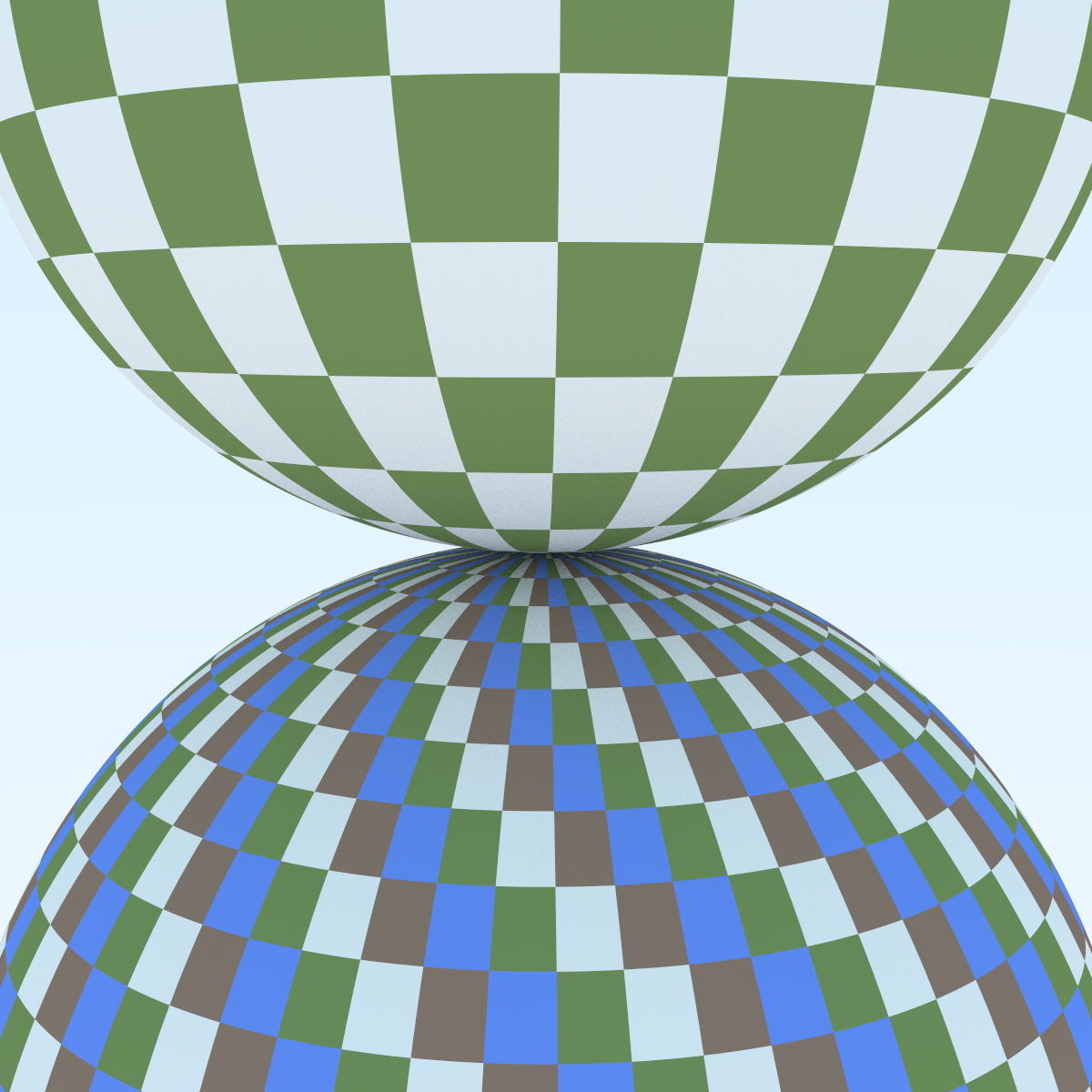scene surf_check_sc() {    hittable_list world;    auto checker1 = make_shared<surface_checker>(        surface_checker::text_array{            make_shared<fixed_color>(color(0.2, 0.3, 0.1)),            make_shared<fixed_color>(color(0.9, 0.9, 0.9)),            make_shared<fixed_color>(color(0.3, 0.2, 0.15)),            make_shared<fixed_color>(color(0.15, 0.3, 0.9))},        std::pair<f8, f8>{60, 60});    auto checker2 = make_shared<surface_checker>(        surface_checker::text_array{            make_shared<fixed_color>(color(0.2, 0.3, 0.1)),            make_shared<fixed_color>(color(0.9, 0.9, 0.9)),        },        std::pair<f8, f8>{30, 30});    world.add(make_shared<sphere>(pt3(0, -10, 0), 10,                                  make_shared<lambertian>(checker1)));    world.add(make_shared<sphere>(pt3(0, 10, 0), 10,                                  make_shared<lambertian>(checker2)));    f8 asp_ratio = 1.0;    vec3 lookfrom = pt3(13, 2, 3) * 2;    vec3 lookat = pt3(0, 0, 0);    f8 vfov = 40.0;    auto dist_to_focus = 10.0;    auto aperture = 0;    vec3 vup(0, 1, 0);    auto cam_ptr = make_shared<camera>(lookfrom, lookat, vup, vfov, asp_ratio,                                       aperture, dist_to_focus, aperture, 1.0);    return scene(make_shared<bvh_node>(world), blue_sky_back_ptr, cam_ptr);}

# 柏林噪声import numpy as npimport matplotlib.pyplot as pltfrom math import *XLEN = 25 # 产生多少个整数点YLEN = 25DIFF = 0.05ptsx = np.arange(0, XLEN, DIFF)ptsy = np.arange(0, YLEN, DIFF)xs, ys = np.meshgrid(ptsx, ptsy)z_orig = np.random.random((XLEN + 1, YLEN + 1))z_interped = np.zeros((round((XLEN) / DIFF), round(YLEN / DIFF)))def lerp(a, b, t):    return a + t * (b - a)def lerp2(ld, rd, lu, ru, tx, ty): # 二维线性插值    # left down, right down, left up, right up    upmid = lerp(lu, ru, tx)    dnmid = lerp(ld, rd, tx)    return lerp(dnmid, upmid, ty)for i in range(XLEN):    for si in range(round(1 / DIFF)):  # step i        for j in range(YLEN):            for sj in range(round(1 / DIFF)):                z_interped[i * round(1 / DIFF) + si][j * round(1 / DIFF) + sj] = lerp2(                    z_orig[i][j], z_orig[i + 1][j], z_orig[i][j + 1],  z_orig[i + 1][j + 1], DIFF * si, DIFF * sj)plt.imshow(z_interped, cmap=plt.cm.gray)plt.savefig("./2d.png", dpi = 150, format = 'png')plt.show()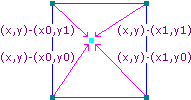$\vec{v_i} = \vec{d_i} \cdot \vec{g_i}$• 红框中大部分是黄色的，因为这部分点的距离向量和关键点的随机向量有相似的方向。
• 黄框中大部分是蓝色的，因为其左下角关键点随机向量的尾部指向了这片区域，也就是这片区域的距离向量和关键点随机向量相反。
• 绿框中的大部分是黄色的，虽然这片区域是左边关键点随机向量的反方向，但因为线性插值的存在，并且这片区域离右边的随机向量更近，其受到右边随机向量的影响更大。

## 湍流（turbulence）

double turb(const point3& p, int depth=7) const {    auto accum = 0.0;    auto temp_p = p;    auto weight = 1.0;    for (int i = 0; i < depth; i++) {        accum += weight*noise(temp_p);        weight *= 0.5;        temp_p *= 2;    }    return fabs(accum);}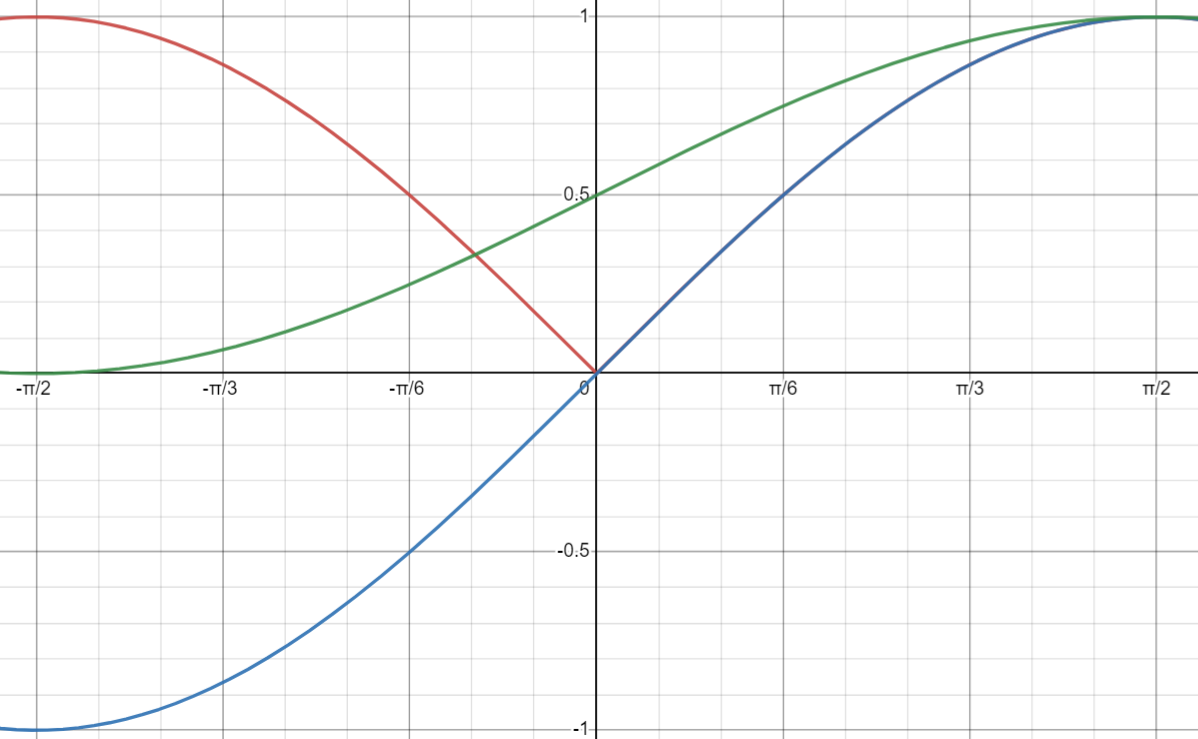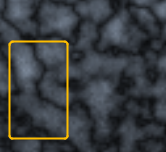### 一些疑问

Noise()
In order to get the most out of the PSE and the solid texture approach we have provided some primitive stochastic functions with which to bootstrap visual complexity. We now introduce the most fundamental of these. Noise() is a scalar valued function which takes a three dimensional vector as its argument. It has the following properties :

• Statistical invariance under rotation (no matter how we rotate its domain, it has the same statistical character)
• A narrow bandpass limit in frequency (its has no visible features larger or smaller than within a certain narrow size range)

Appendix. Turbulence
A suitable procedure for the simulation of turbulence using the Noise() signal is :

function turbulence(p)  t = 0  scale = 1  while (scale > pixelsize)      t += abs(Noise(p / scale) * scale)      scale /= 2  return t

By evaluating Noise() at visible surface points of simulated objects we may create a simple “random” surface texture (figure Spotted.Donut) :
color = white * Noise(point)

# 实例变换

## 旋转矩阵

### 公式推导

$x^\prime = \cos(\theta) - \sin(\theta) \cdot y \\y^\prime = \sin(\theta) + \cos(\theta) \cdot y$

$x = r\cos\phi \\y = r\sin\phi$

$x^\prime = r\cos(\phi + \theta) \\y^\prime = r\sin(\phi + \theta)$

$\cos(\phi + \theta) = \cos\phi\cos\theta - \sin\phi\sin\theta \\\sin(\phi + \theta) = \sin\phi\cos\theta + \cos\phi\sin\theta$

\begin{align*} x^\prime &= r(\cos\phi\cos\theta - \sin\phi\sin\theta) \\ x^\prime &= (r\cos\phi)\cos\theta - (r\sin\phi)\sin\theta \\ x^\prime &= x\cos\theta - y\sin\theta \end{align*}

\begin{align*} y^\prime &= r(\sin\phi\cos\theta + \cos\phi\sin\theta) \\ y^\prime &= r(\sin\phi)\cos\theta + r(\cos\phi)\sin\theta \\ y^\prime &= y\cos\theta + x\sin\theta \\ &= x\sin\theta + y\cos\theta\end{align*}

### 一些解释

$x$ 轴的旋转基本和这个没区别，但是绕 $y$ 轴的比较令人疑惑了。

           y+           |           |            |x- ------- z --------- x+           |           |           |           y-

           y+           |           |            |z+ ------- x --------- z-           |           |           |           y-

\begin{align*} y^\prime &= \cos \theta \cdot y - \sin\theta \cdot z \\ &= \cos(90) \cdot 1 - \sin(90) \cdot 0 \\ &= 0 - 0 = 0\end{align*}

\begin{align*} z^\prime &= \sin \theta \cdot x + \cos \theta \cdot z \\ &= \sin(90) \cdot 1 + \cos(90) \cdot z \\ &= 1 + 0 = 1\end{align*}

           z-           |           |            |x- ------- y --------- x+           |           |           |           z+

\begin{align*} z^\prime &= \sin \theta \cdot x + \cos \theta \cdot z \\ &= \cos(90) \cdot 0 - \sin(90) \cdot -1 \\ &= 0 - (1 \cdot -1) = 1\end{align*}

# 多线程

]]>
<link rel="stylesheet" type="text/css" href="https://cdn.jsdelivr.net/hint.css/2.4.1/hint.min.css"><p>一个多月终于断断续续搞完了第二本书里的内容。和前面两篇文章一样，这篇也会写一些我个人花了较长时间才搞懂的部分，以及一些我在原书基础上加的新功能。</p> <p>对于原书就有的功能，会直接使用书上的代码，如果是我新加进去的功能，会使用自己的代码。因为我的代码在原书基础上做了较大幅度的变化（即使是原来就有的功能），所以只看一段代码可能不太明白，这里可以参考我的 GitHub 仓库： <a
Ray Tracing in One Weekend 学习笔记（2）：相机类的实现 https://ttzytt.com/2022/09/RTOW_note2/ 2022-09-06T04:00:00.000Z 2022-09-07T03:37:25.044Z

# 相机类的实现

## 相机的定位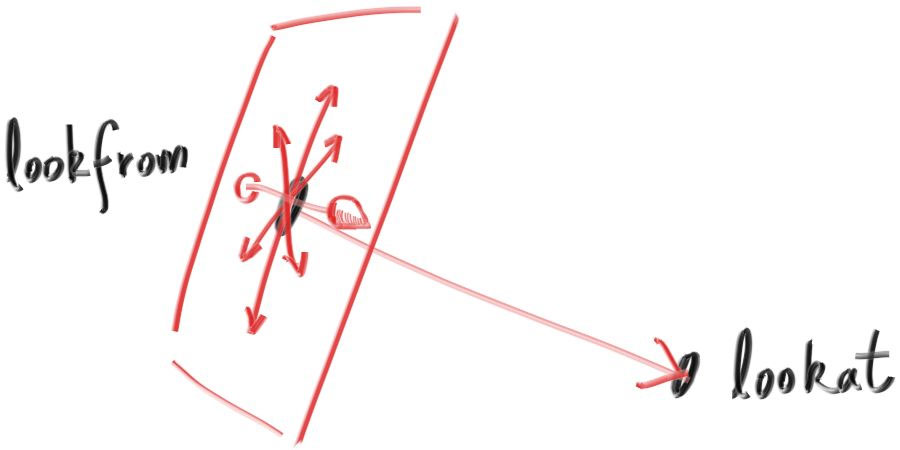#pragma once#include "rtow.h"// 这里的 f8 就是 double （八个字节的 float）class camera {   public:    camera(vec3 lookfrom, vec3 lookat, vec3 vup = vec3(0, 1, 0), f8 vfov = 90,           f8 asp_ratio = 16.0 / 9.0, f8 aperture = 0, f8 foc_len = 1) {        f8 deg_fov = deg2rad(vfov);        f8 half_hei = tan(deg_fov / 2);  // 对边比临边，但是临边是 1        f8 half_wid = half_hei * asp_ratio;        cam_z = (lookfrom - lookat).unit_vec();        // z 和镜头指向位置是反的        cam_x = cross(vup, cam_z).unit_vec();  // 同时和 vup，z 垂直        cam_y = vup.unit_vec();        horizon = 2 * half_wid * cam_x * foc_len; // 焦平面的横竖边框        vertic = 2 * half_hei * cam_y * foc_len;        orig = lookfrom;        lower_left_corner = orig - horizon / 2 - vertic / 2 - cam_z * foc_len; // 焦平面的左下角        len_radius = aperture / 2;    }    inline ray get_ray(f8 x, f8 y) const {        // x 和 y 的范围：[0, 1]        // 相机传感器的像素点坐标        vec3 rd = len_radius * rand_unit_disk();        vec3 offset = cam_x * rd.x() + cam_y * rd.y();         ray r;        r.orig = orig + offset;        r.dir = lower_left_corner + x * horizon + y * vertic -                orig - offset;              // 产生一个从 orig + offset 到对应像素的向量            // 因为 ray 对应的就是 orig + t * dir        return r;    }    vec3 orig;               // 摄像机的位置    vec3 lower_left_corner;  // 画面的左下角    vec3 horizon, vertic;    // 画面的尺寸（或者说离相机 foc_len 的平面大小）    vec3 cam_x, cam_y, cam_z;// 相机朝向    f8 len_radius;           // 光圈半径};f8 deg_fov = deg2rad(vfov);f8 half_hei = tan(deg_fov / 2);  // 对边比临边，但是临边是 1f8 half_wid = half_hei * asp_ratio;

cam_z = (lookfrom - lookat).unit_vec();// z 和镜头指向位置是反的cam_x = cross(vup, cam_z).unit_vec();  // 同时和 vup，z 垂直cam_y = vup.unit_vec();
• cam_z 表示一个从 lookatloofrom 的方向，这个方向和相机实际拍摄的位置是相反的。
• cam_x 的计算用到了向量的叉乘，在三维空间中，如果 $u = v \times w$ 那么 $u$ 就是同时垂直于 $v$$w$ 的，当然符合这个条件的向量有两个，可以用右手定则确定，这里就不赘述了。根据前面的这个定义，可以得出 cam_x 同时和 cam_zvup（也就是 cam_z） 垂直。
• cam_y 就是 vup 的单位向量。

https://www.cnblogs.com/qilinzi/archive/2013/05/09/3068158.html

## 相机景深的实现

### 现实中的景深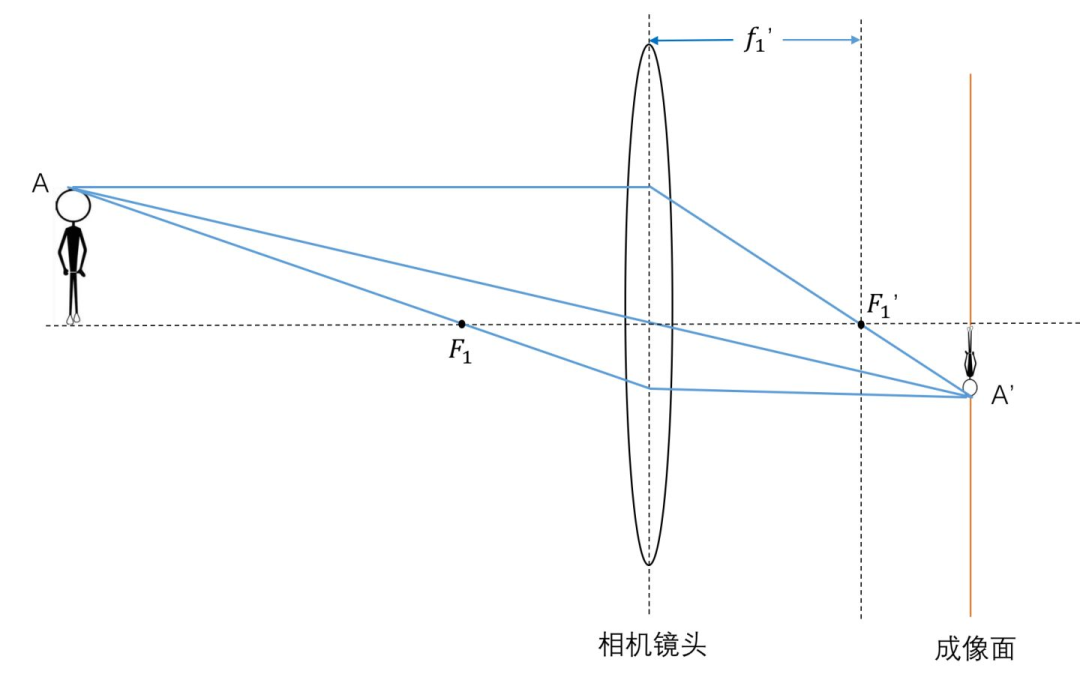1. 同一点发出的各个方向的光线，经过镜头后必定汇聚于同一点
2. 同一平面上的不同点发出的光线，经过镜头后，汇聚于不同点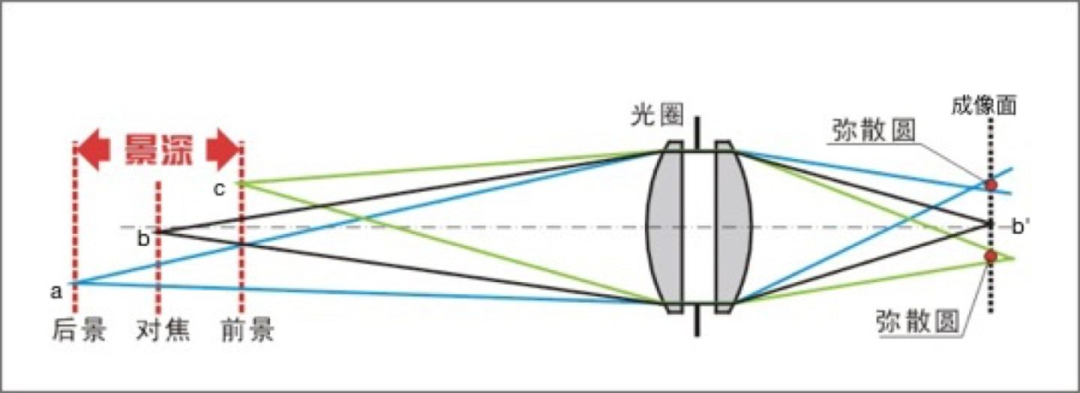### 实际实现1. https://jishuin.proginn.com/p/763bfbd2e03f
]]>
<link rel="stylesheet" type="text/css" href="https://cdn.jsdelivr.net/hint.css/2.4.1/hint.min.css"><h1>相机类的实现</h1> <p>除了朗伯体，RTOW 中还有个比较有趣的地方就是相机类的实现，特别是背景虚化这部分。</p> <h2
Ray Tracing in One Weekend 学习笔记（1）：朗伯体和辐射度量学 https://ttzytt.com/2022/08/RTOW_note1/ 2022-08-31T04:00:00.000Z 2022-09-07T03:37:20.191Z

# 朗伯体材质 （Lambertian） 的实现

class lambertian : public material {   public:    lambertian(const color& alb) : albedo(alb) {}        virtual optional<pair<ray, color>>     get_ray_out(const ray& r_in, const hit_rec& rec) const override {        vec3 ref_dir = rec.norm + rand_unit_vec(); // 注意这里        if(ref_dir.near_zero()) // 如果 rand_unit_vec() 等于 -rec.norm             ref_dir = rec.norm;        ray ref_ray(rec.hit_pt, ref_dir);        return make_pair(ref_ray, albedo);        }    color albedo;  // 反射率};

## 辐射度量学

### 基本单位

$\Phi = \frac{\mathrm{d}Q}{\mathrm{d}t}$

$E = \frac{\rm{d}\Phi}{\rm{d}A}$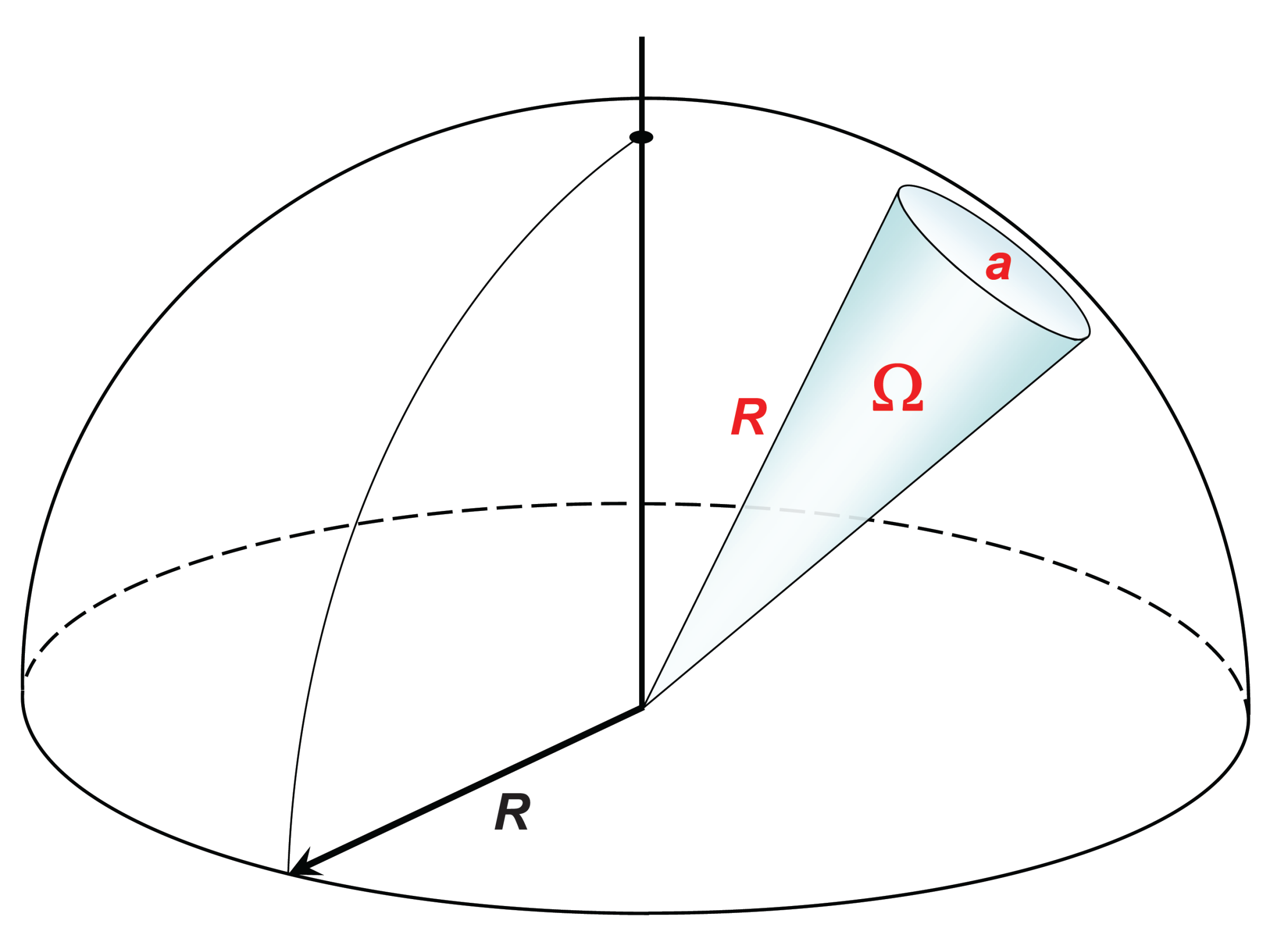$\Omega = \frac{a}{R^2}$

$L_\theta = \frac{\rm{d} \Phi}{\rm{d}A\cos(\theta)\rm{d}\Omega}$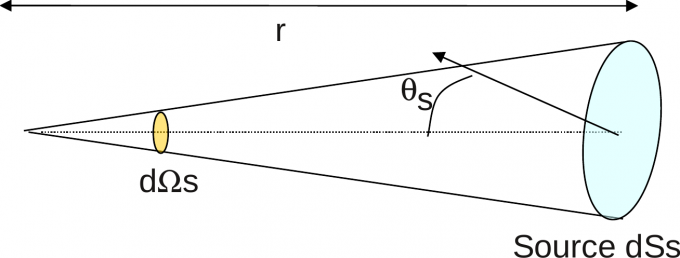$I = \frac{\rm{d}\Phi}{\rm{d}\Omega}$

### 朗伯余弦定理$I_{\theta} = I_n \times \cos \theta$

## 朗伯体和漫反射

$I_{\theta} = I_n \times \cos \theta$

$L_\theta = \frac{\rm{d} \Phi}{\rm{d}A\cos(\theta)\rm{d}\Omega}$

$I_{\theta} = \frac{\rm{d}\Phi}{\rm{d}\Omega} \cos \theta$

$L_\theta = \frac{I_n \cancel{\cos \theta}}{\rm{d}A\cancel{\cos\theta}}$

## 所以，代码为什么这么写？

……color pixel_color(0, 0, 0);for (int s = 0; s < samples_per_pixel; ++s) {    auto u = (i + random_double()) / (image_width-1);    auto v = (j + random_double()) / (image_height-1);    ray r = cam.get_ray(u, v);    pixel_color += ray_color(r, world);}write_color(std::cout, pixel_color, samples_per_pixel);……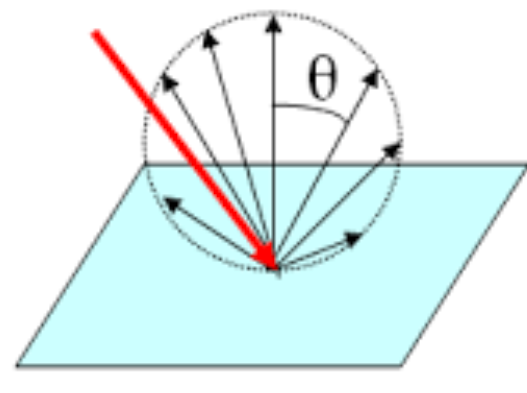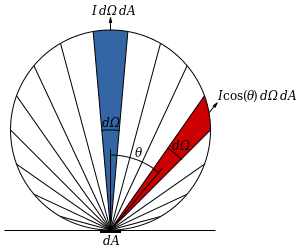vec3 ref_dir = rec.norm + rand_unit_vec();

$I_{\theta} = I_n \times \cos \theta$]]>
<link rel="stylesheet" type="text/css" href="https://cdn.jsdelivr.net/hint.css/2.4.1/hint.min.css"><p><s>最近</s>（距离搞完 RTOW 已经过去一周了，我现在才把这笔记写出来，属实是懒狗）花了一些时间看完了 Ray Tracing in One Weekend （以下简称 RTOW）<s>果然还是我太菜了，这玩意 One Weekend
[MIT 6.s081] Xv6 Lab11 Mmap 实验记录 https://ttzytt.com/2022/08/xv6_lab11_record/ 2022-08-21T04:00:00.000Z 2022-10-15T22:48:49.466Z

upd@2022/9/14：最近把实验的代码放到 github 上了，如果需要参考可以查看这里：

https://github.com/ttzytt/xv6-riscv

# Lab11: mmap

## 描述

mmap() 的定义如下：

void *mmap(void *addr, size_t length, int prot, int flags,           int fd, off_t offset);

protflags 都是一些标志位，具体说，prot 有以下的选项：

• PROT_NONE
• PROT_WRITE
• PROT_EXEC

flags 则决定，如果在内存映射文件中做了修改，是否要在取消映射时，把这些修改更新到文件中。

unmap() 的定义如下：

int munmap(void *addr, size_t length);

## 整体思路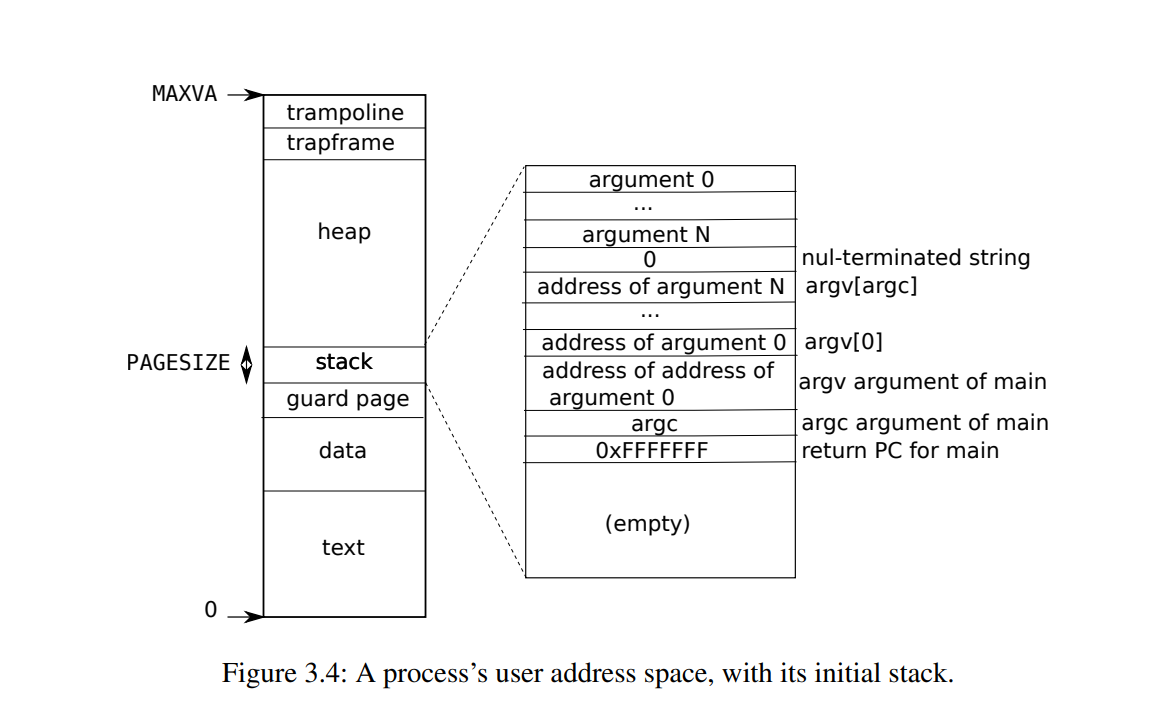uint64sys_sbrk(void){  int addr;  int n;  if(argint(0, &n) < 0)    return -1;  addr = myproc()->sz;  if(growproc(n) < 0)    return -1;  return addr;}

## 代码

struct mmap_vma

// in proc.hstruct mmap_vma{  int in_use;      // 该 vma 结构体是否代表了一个正在使用的文件映射  uint64 sta_addr; // 起始地址  uint64 sz;       // 映射大小  int prot;  struct file* file; // 映射的文件  int flags;         // map_shared or map_private};#define VMA_SZ 16struct proc {  ……  struct mmap_vma mmap_vams[VMA_SZ];}

sys_mmap()

// in sysfileuint64 sys_mmap(){  uint64 addr, length, offset; // addr 和 offset 都只有 0  int prot, flags, fd;  struct file* file;  //void *mmap(void *addr, size_t length, int prot, int flags, int fd, off_t offset);  // 这参数是真的多。。  try(argaddr(0, &addr), return -1)  try(argaddr(1, &length), return -1)  try(argint(2, &prot), return -1)  try(argint(3, &flags), return -1)  try(argfd(4, &fd, &file), return -1) // 同时取得文件和描述符  try(argaddr(5, &offset), return -1)  // 读入参数  struct proc* p = myproc();  if(addr || offset) // 我们实现的是 mmap 的子集，不支持自定内存和偏移量    return -1;  if(!file->writable && (prot & PROT_WRITE) && (flags & MAP_SHARED))    return -1;  // 如果文件本身不允许写入，但 PROT_WRITE 还是设置了  int unuse_idx = -1;  uint64 sta_addr = get_mmap_space(length, p->mmap_vams, &unuse_idx);  if(unuse_idx == -1)    return -1;  if(sta_addr <= p->sz) // 没内存来 mmap 了    return -1;  struct mmap_vma* cur_vma = &p->mmap_vams[unuse_idx];  cur_vma->file = file;  cur_vma->in_use = 1;  cur_vma->prot = prot;  cur_vma->flags = flags;  cur_vma->sta_addr = sta_addr;   cur_vma->sz = length;  filedup(file); // 增加引用计数  return cur_vma->sta_addr;} 

get_mmap_space()// in sysfile.cuint64get_mmap_space(uint64 sz, struct mmap_vma* vmas, int* free_idx){  *free_idx = -1;    // 返回一个可以储存新文件映射的地址（开始地址）  // 优先查看 vma 槽中的“空隙”，如果没有，那就映射到最下面  // 其实可以写一个快速排序，但是我懒。。。  uint64 lowest_addr = TRAPFRAME;    struct mmap_vma tmp; // 作为上边界，可能和上图一样，最上方没有任何映射区域  tmp.sta_addr = TRAPFRAME, tmp.sz = 0;  for(int i = 0; i <= VMA_SZ; i++){    // 假设 vmas[i] 的 PGROUNDDOWN(sta_addr) 是新文件映射的结束位置    if(vmas[i].in_use == 0 && i != VMA_SZ){      *free_idx = i;      continue;    }     uint64 ed_pos = i != VMA_SZ ? PGROUNDDOWN(vmas[i].sta_addr)                                 : tmp.sta_addr;    lowest_addr = ed_pos < lowest_addr ? ed_pos : lowest_addr; // 取 min        for(int j = 0; j < VMA_SZ; j++){      // 假设 vmas[j] 的 sta_addr + sz（vma[j] 的结束位置） 往上是新映射的起始位置      if(vmas[j].in_use == 0 && i != VMA_SZ) continue;      uint64 st_pos = i != VMA_SZ ? vmas[j].sta_addr + vmas[j].sz                                   : tmp.sta_addr + tmp.sz; // 这个位置一定是页对齐的                                        if (ed_pos <= st_pos) continue;       // 这里直接跳过，不在下面判断是因为无符号类，如果做下面的减法会出错      if (ed_pos - st_pos >= sz){        // [st_pos, ed_pos) 的区间        return st_pos;      }    }  }   return lowest_addr - sz;}

mmap_fault_handler()

// in trap.cint mmap_fault_handler(uint64 addr){  struct proc* p = myproc();  struct mmap_vma* cur_vma;  if((cur_vma = get_vma_by_addr(addr)) == 0){    // 找到这个地址属于哪个文件的映射    // 等于零说明不属于任何一个    return -1;  }  if(!cur_vma->file->readable && r_scause() == 13 && cur_vma->flags & MAP_SHARED){    DEBUG("mmap_fault_handler: not readable\n");    return -1;  } // 读错误      if(!cur_vma->file->writable && r_scause() == 15 && cur_vma->flags & MAP_SHARED){    DEBUG("mmap_fault_handler: not writable\n");    return -1;  } // 写错误      uint64 pg_sta = PGROUNDDOWN(addr);  uint64 pa = kalloc();  if(!pa){    DEBUG("mmap_fault_handler: kalloc failed\n");    return -1;  }  memset(pa, 0, PGSIZE);  int perm = PTE_U | PTE_V;  if(cur_vma->prot & PROT_READ) perm |= PTE_R;  if(cur_vma->prot & PROT_WRITE) perm |= PTE_W;  if(cur_vma->prot& PROT_EXEC) perm |= PTE_X;  // 在 mmap 的时候已经排除了不可能的情况了  uint64 off = PGROUNDDOWN(addr - cur_vma->sta_addr);   // 这个 off 代表文件拷贝时要跳过多少个页帧  ilock(cur_vma->file->ip);  int rdret;  if((rdret = readi(cur_vma->file->ip, 0, pa, off, PGSIZE)) == 0){    iunlock(cur_vma->file->ip);    return -1;  }  iunlock(cur_vma->file->ip); // 没有 put 是这个文件之后还需要使用                              // 在 unmap 中应该可以 put  mappages(p->pagetable, pg_sta, PGSIZE, pa, perm);  return 0;}

get_vma_by_addr()

struct mmap_vam* get_vma_by_addr(uint64 addr){  struct proc* p = myproc();  for(int i = 0; i < VMA_SZ; i++){    if(p->mmap_vams[i].in_use && addr >= p->mmap_vams[i].sta_addr && addr < p->mmap_vams[i].sta_addr + p->mmap_vams[i].sz){      // 判断该地址是否在文件映射区的中间      return p->mmap_vams + i;    }  }  return 0;}

usertrap()

// in trap.c……if(r_scause() == 8){  // system call  if(p->killed)    exit(-1);  // sepc points to the ecall instruction,  // but we want to return to the next instruction.  p->trapframe->epc += 4;  // an interrupt will change sstatus &c registers,  // so don't enable until done with those registers.  intr_on();  syscall();} else if((which_dev = devintr()) != 0){  // ok} else if ((r_scause() == 13 || r_scause() == 15)){  try(mmap_fault_handler(r_stval()), bad = 1)}else{  bad = 1;}if (bad){  printf("usertrap(): unexpected scause %p pid=%d\n", r_scause(), p->pid);  printf("            sepc=%p stval=%p\n", r_sepc(), r_stval());  p->killed = 1;}……

#define PTE_D (1L << 7)

• unmap 的部分可能没有跨过一个页帧，也就是说 unmap 的所有内存都在一个页帧之内，那么这个页帧不能释放，但是需要把内存中的数据复制回文件。
• 对于 unmap 结尾地址在某个页帧中间的情况，需要分类讨论。如果结尾是中间，但这个页帧是映射区的最后一个页帧，那既需要释放页帧，也许要写回文件。如果是中间，但不是最后一个页帧，那就不能释放。

// in vm.cintmmap_writeback(pagetable_t pt, uint64 src_va, uint64 len, struct mmap_vma* vma){// 把带脏位的页帧写回文件中，并且取消映射// 写回的是 src_va 开始的，长度为 len  uint64 a;  pte_t *pte;  for(a = PGROUNDDOWN(src_va); a < PGROUNDDOWN(src_va + len); a += PGSIZE){    if((pte = walk(pt, a, 0)) == 0){       panic("mmap_writeback: walk");    }    if(PTE_FLAGS(*pte) == PTE_V)      panic("mmap_writeback: not leaf");    if(!(*pte & PTE_V)) continue; // 懒分配    if((*pte & PTE_D) && (vma->flags & MAP_SHARED)){       // 写回      begin_op();      ilock(vma->file->ip);      uint64 copied_len = a - src_va;      writei(vma->file->ip, 1, a, copied_len, PGSIZE);      iunlock(vma->file->ip);      end_op();    }    kfree(PTE2PA(*pte));    *pte = 0;  }  return 0;}

//in vm.cintmmap_writeback_na(pagetable_t pt, uint64 src_va, uint64 len, struct mmap_vma* vma){  uint64 a;  pte_t *pte;  a = PGROUNDDOWN(src_va);  if(a == PGROUNDDOWN(src_va + len)){     // 如果 unmap 的部分在一个页帧的范围内    begin_op();    ilock(vma->file->ip);    writei(vma->file->ip, 1, src_va, 0, src_va - a);    iunlock(vma->file->ip);    end_op();  }  for(; a < PGROUNDDOWN(src_va + len); a += PGSIZE){ // 这部分只处理整页                                                     // 如果结尾停在页中间不会处理    if((pte = walk(pt, a, 0)) == 0){       panic("mmap_writeback: walk");    }    if(PTE_FLAGS(*pte) == PTE_V)      panic("mmap_writeback: not leaf");    if(!(*pte & PTE_V)) continue; // 懒分配    if((*pte & PTE_D) && (vma->flags & MAP_SHARED)){       // 写回      begin_op();      ilock(vma->file->ip);      // 第一次的时候，a 会比 src_va 小      uint64 copied_len = a - src_va;      if(a < src_va){         // 第一个页帧，不是完整的        // 这种情况也需要 kfree，因为跨过了一个页帧        writei(vma->file->ip, 1, src_va, 0, src_va - a);       } else {        writei(vma->file->ip, 1, a, copied_len, PGSIZE);      }       iunlock(vma->file->ip);      end_op();    }    kfree(PTE2PA(*pte));    *pte = 0;  }    uint64 copied_len = a - src_va;  uint64 len_left = vma->sz - copied_len;  if (len_left){    // 处理 unmap 结尾在页帧中间的情况    begin_op();    ilock(vma->file->ip);    writei(vma->file, 1, a, copied_len, len_left);    if(len_left + a == vma->sz + src_va){ // 如果停在的页帧刚好是最后一个      pte_t *pte;      if((pte = walk(pt, a, 0)) == 0){         panic("mmap_writeback: walk");      }      kfree(PTE2PA(*pte));    }    iunlock(vma->file->ip);    end_op();  }  return 0;}

// in sysfile.cuint64munmap(uint64 addr, uint64 len){  struct proc* p = myproc();  struct mmap_vma* cur_vma = get_vma_by_addr(addr);  if(!cur_vma)    return -1;  if(addr > cur_vma->sta_addr && addr + len < cur_vma->sta_addr + cur_vma->sz){    // 从中间挖洞    return -1;  }  mmap_writeback(p->pagetable, addr, len, cur_vma);   if(addr == cur_vma->sta_addr){     // 从起始位置删除的    cur_vma->sta_addr += len;  }   cur_vma->sz -= len;    if(cur_vma->sz <= 0){    // 如果整个映射区都没了    fileclose(cur_vma->file);    cur_vma->in_use = 0;  }  return 0;  }

uint64sys_munmap(){  // int munmap(void *addr, size_t length);  uint64 addr;  uint64 len;  try(argaddr(0, &addr),  return -1)  try(argaddr(1, &len), return -1)  return munmap(addr, len);}

// in proc.c wait():……  for(;;){    // Scan through table looking for exited children.    havekids = 0;    for(np = proc; np < &proc[NPROC]; np++){      if(np->parent == p){        // make sure the child isn't still in exit() or swtch().        acquire(&np->lock);        havekids = 1;        if(np->state == ZOMBIE){          // Found one.          pid = np->pid;          if(addr != 0 && copyout(p->pagetable, addr, (char *)&np->xstate,                                  sizeof(np->xstate)) < 0) {            release(&np->lock);            release(&wait_lock);            return -1;          }          freeproc(np); // 注意这里，只有父进程 wait 的时候才会去 freeproc。          release(&np->lock);          release(&wait_lock);          return pid;        }        release(&np->lock);      }    }    ……  }……

// in proc.c exit():voidexit(int status){  struct proc *p = myproc();  if(p == initproc)    panic("init exiting");  // 释放和写回 mmap 数据需要在关闭文件之前  for(int i = 0; i < VMA_SZ; i++){    if(p->mmap_vams[i].in_use){      try(munmap(p->mmap_vams[i].sta_addr, p->mmap_vams[i].sz), panic("exit: munmap"));    }  }  // Close all open files.  for(int fd = 0; fd < NOFILE; fd++){    if(p->ofile[fd]){      struct file *f = p->ofile[fd];      fileclose(f);      p->ofile[fd] = 0;    }  }……}

fork()

// in proc.c……  for (int i = 0; i < VMA_SZ; i++){    if(p->mmap_vams[i].in_use){      np->mmap_vams[i] = p->mmap_vams[i];       filedup(p->mmap_vams[i].file);      // 复制 vma    }  }……

// in vm.c  for(i = 0; i < sz; i += PGSIZE){ // 注意这里范围    if((pte = walk(old, i, 0)) == 0)      panic("uvmcopy: pte should exist");    if((*pte & PTE_V) == 0)      panic("uvmcopy: page not present");    pa = PTE2PA(*pte);    flags = PTE_FLAGS(*pte);    if((mem = kalloc()) == 0)      goto err;    memmove(mem, (char*)pa, PGSIZE);    if(mappages(new, i, PGSIZE, (uint64)mem, flags) != 0){      kfree(mem);      goto err;    }  }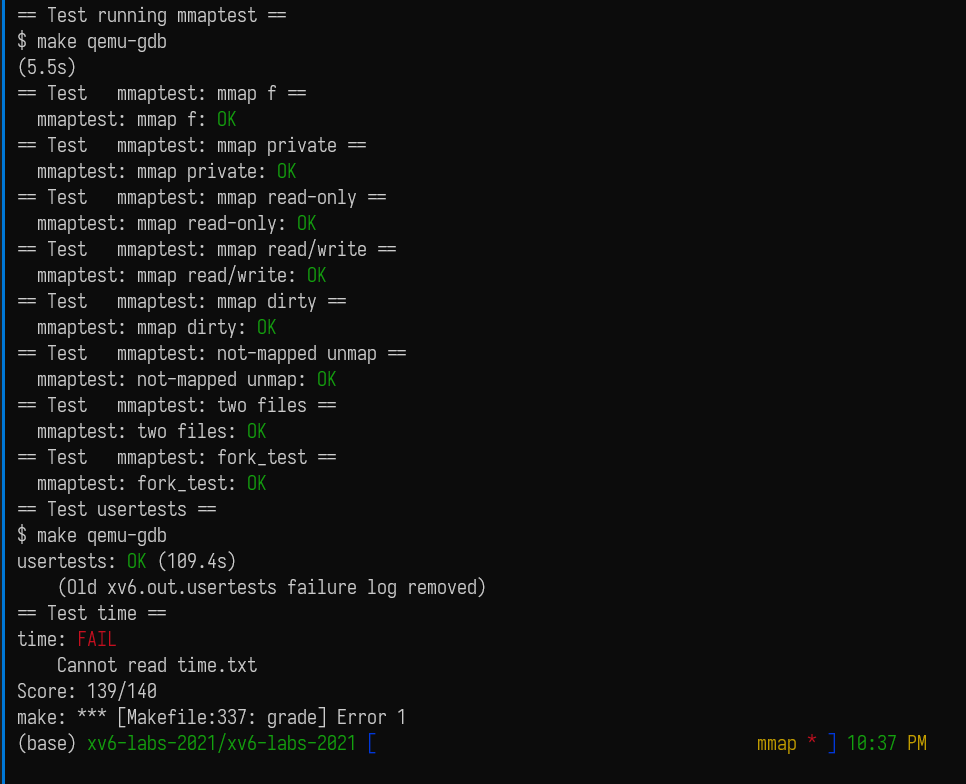## 吐槽

CFLAGS = -Wall -O -g3 -fno-omit-frame-pointer -ggdb -UFDEBUG

### 思路

struct {  struct spinlock lock;  struct run *freelist;} kmems[NCPU];void kalloc(){    struct run* r = 0;    push_off();    int cpu = cpuid();    pop_off();    acquire(&kmems[cpu].lock);    int stealed = 0;    if(!kmems[cpu].freelist){        for (i : kmems){            acquire(&i.lock);            while (i 中还有页帧 && stealed < STEAL_CNT) {                释放 i 中 feelist 的页帧;                把释放的页帧加入 kmems[cpu].freelist;            }            if(stealed >= STEAL_CNT){                break;            }            releae(&i.lock);        }    }    r = kmems[cpu].freelist;    if (r) {            kmems[cpu].freelist = r->next;    }    release(&kmems[cpu].lock);    return r;}

### 代码

kinit()

struct {  struct spinlock lock, stlk;  struct run *freelist;  uint64 st_ret[STEAL_CNT]; // 候选队列} kmems[NCPU];const uint name_sz = sizeof("kmem cpu 0");char kmem_lk_n[NCPU][sizeof("kmem cpu 0")];voidkinit(){   for(int i = 0; i < NCPU; i++){    snprintf(kmem_lk_n[i], name_sz, "kmem cpu %d", i);    initlock(&kmems[i].lock, kmem_lk_n[i]);  }  freerange(end, (void*)PHYSTOP);}

kfree()

voidkfree(void *pa){  struct run *r;  if(((uint64)pa % PGSIZE) != 0 || (char*)pa < end || (uint64)pa >= PHYSTOP)    panic("kfree");  push_off();  uint cpu = cpuid();  pop_off();  // Fill with junk to catch dangling refs.  memset(pa, 1, PGSIZE);  r = (struct run*)pa;  acquire(&kmems[cpu].lock);  r->next = kmems[cpu].freelist;  kmems[cpu].freelist = r;  release(&kmems[cpu].lock);}

steal()

int steal(uint cpu){ // 返回偷到了几个  uint st_left = STEAL_CNT;  int idx = 0;   memset(kmems[cpu].st_ret, 0, sizeof(kmems[cpu].st_ret));  for(int i = 0; i < NCPU; i++){    if(i == cpu)  continue;    acquire(&kmems[i].lock);    while(kmems[i].freelist && st_left){       kmems[cpu].st_ret[idx++] = kmems[i].freelist;        kmems[i].freelist = kmems[i].freelist->next;        st_left--;    }    release(&kmems[i].lock);    if(st_left == 0) { // 一共偷 STEAL_CNT 个      break;    }  }  return idx;}

kalloc()

void *kalloc(void){  struct run *r = 0;    push_off();  uint cpu = cpuid();     acquire(&kmems